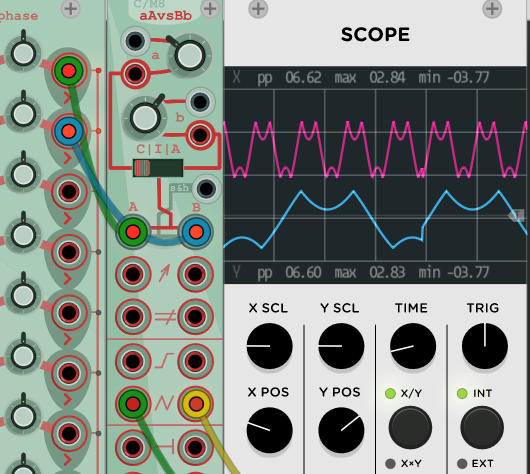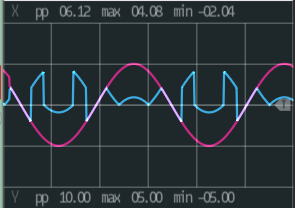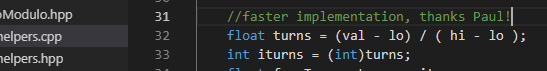# Trying to make a wavefolder but it freezes Rack

I’m trying to implement a wave folding function for a module, and generally the code works, but when I reset the 2 parameters that get passed as the lo and hi variables, the module hangs and sometimes Rack freezes.

I understand with very small ranges, the following code is bound to be slow because it keeps folding the value around the upper and lower limit until it falls within that limit.

What I don’t get is why it just stutters when I set lo and hi close together, but freezes alltogether when both are set to exactly the same value (even though I check for that in my code). Also, sometimes it does not freeze at allThis is the function:

``````
float cm_fold(float val, float lo = -10.0, float hi = 10.0){
lo = (lo * 0.1) * 10;
hi = (hi * 0.1) * 10;
if (lo == hi){
return lo;
}else if (lo < hi){
while (val < lo || val > hi){
if (val < lo){
val = -(val - lo) + lo;
}else if (val > hi){
val = hi + (hi - val);
}
}
return val;
}
return 0.0;
}
``````

and it’s called in step():

``````void CM8Module::step() {

//set limits
lo = (inputs[INPUT__a].active) ? (inputs[INPUT__a].value * 0.1) * params[PARAM__a].value : params[PARAM__a].value;
hi = (inputs[INPUT__b].active) ? (inputs[INPUT__b].value * 0.1) * params[PARAM__b].value : params[PARAM__b].value;

//A ONLY
if (inputs[INPUT_A].active) outputs[OUTPUT_AFLD].value = cm_fold(inputs[INPUT_A].value, lo, hi);

//B ONLY
if (inputs[INPUT_B].active) outputs[OUTPUT_BFLD].value = cm_fold(inputs[INPUT_B].value, lo, hi);

}
``````

I’m pretty sure there must be a more performant way of doing this, but google only serves me results about folding dsp architectures and folding in c++, none of which have anything to do with folding values around a rangeSo 2 questions actually: why would this happen? And is there a better way to do this?Is this what you want? (edited)
(just a guess, didn’t test the code)

``````float cm_fold(float val, float lo, flow hi) {
float range = hi - lo;
float phase = (val - lo) % (range * 2);
if (phase < 0) {
phase += range * 2;
}
return lo + (phase > range ? range * 2 - phase : phase);
}``````

note really, when using that, for example cm_fold(4.1, 2.0, 3.0); will return 2.0 instead of 2.1, which is like a clamp with 1 fold.

So what it has to do is this:Which is all good, but when I reset both parameters I get this:

The problem is that your while loop can be a really inefficient way to find the range. For instance, with val = 13, lo = 0.01 and hi = 0.07 it takes 216 iterations to get to 0.04 and, in that time, has accumulated errors at the 4th decimal place. I think basically what happens in your code is you run into a pathological case where your while loop runs for a very very long time. (Basically your while loop is doing val = - val + 2 * hi; or val = -val + 2 * lo. That’s a very inefficient way to do a search). So you need some constant time way to do it.

But that’s pretty easy. Basically what you are doing is a wraparound round.

``````    float turns = (val - lo) / ( hi - lo );
int iturns = (int)turns;
float fracTurn = turns - iturns;
if( iturns % 2 )
fracTurn = 1.0 - fracTurn;
float res = (hi-lo) * fracTurn + lo;
``````

For me, this gives the same answer as your while loop, but also has the laudable property of not having unbounded iterations as lo and hi approach each other.

Oh and that should work for negative val I think but I didn’t test it. So you know check edge cases and stuff. The key idea is you are stepping in steps of hi-lo so do it as a jump rather than iteratively

Awesome! That’s exactly the kind of thing I was hoping to find. I knew iterating would be unwise I just didnt find out how to math my way to it otherwiseWill try it out right awayCool.

If it helps to test out all the edge cases, here’s the dumb little standalone C++ program I used to confirm

``````#include <iostream>

int main( int argc, char **argv )
{
float lo, hi, val;
float val0 = 12.9;
lo = -0.2, hi = 0.371, val = val0;
//   lo = 0, hi = 1, val = val0;

for( int i=0; i<10; ++i )
{
val = val0 + i * 0.3;
std::cout << "VALStart= " << val ;
int iterations = 0;
while (val < lo || val > hi) {
iterations ++;
if (val < lo){
val = -(val - lo) + lo;
} else if (val > hi) {
val = hi + (hi - val);
}
}
std::cout << " L=" << lo << " H=" << hi << " V=" << val << " it=" << iterations << std::endl;
}

for( int i=0; i<10; ++i )
{
val = val0 + i * 0.3;
float turns = (val - lo) / ( hi - lo );
int iturns = (int)turns;
float fracTurn = turns - iturns;
if( iturns % 2 )
fracTurn = 1.0 - fracTurn;
float res = (hi-lo) * fracTurn + lo;
std::cout << "VALStart= " << val << " ";
std::cout << "TURNS=" << turns ;
std::cout << " FT=" << fracTurn << " RES=" << res << std::endl;
}
}
``````

Hmm it seems to give some strange results, for exaple with lo of 0.0 and hi of 2.0:

This is with the iterations:And this is with the new code:It looks like strange things happen when the signal goes below the low treshold.
But I get that the idea is to calculate how many “flips” it will have to make in 1 step and cast to int to obtain the fraction to get from the range and then offset it again by the lo value. Which indeed looks like it should work, I dont get how it ends up with values outside of the range and these sudden jumps

OK hold on let me look.

When you are below low fracTurn goes negative. Which isn’t what you want. So you need to increment it and decrement iTurns. Here is the corrected also which matches your iteration in every range I trued -5 to 5 or so with hi lo at 0 2

``````    float turns = (val - lo) / ( hi - lo );
int iturns = (int)turns;
float fracTurn = turns - iturns;
if( fracTurn < 0 )
{
fracTurn = fracTurn + 1;
iturns = iturns - 1;
}
if( iturns % 2 )
fracTurn = 1.0 - fracTurn;
float res = (hi-lo) * fracTurn + lo;
``````

Yes that works as advertised! Meanwhile I got an idea that was so obvious but I forgot to think about simply limiting the iterations to a fixed number and clamp the result values to get rid of errors at near 0 range. It also works and seems fast enough.``````    if (lo == hi){
return lo;
}else if (lo < hi){
int i = 0;
while ((val < lo || val > hi) && i < 100){
i++;
if (val < lo){
val = -(val - lo) + lo;
}else if (val > hi){
val = hi + (hi - val);
}
}
val = std::max(lo, std::min(val, hi));
return val;
}
return 0.0;
``````

@baconpaul’s closed form will be much faster still than 100 iterations of that.

1 Like1 Like

The Squinky Labs “Shaper” module has a wave folder that does not iterate, doesn’t even divide. It’s like the code above. Why don’t you check on a scope and see if what it does it what you want? If so, it’s a function called AudioMath::fold(float). Oh - but perhaps you already got a good one. In that case, never mindWell it divides onceBut yeah that’s a clean implementation for fixed hi/lo! I think where you divide by 2 is the same as where I divide by (hi-lo) since you are setting hi and lo to +1/-1. How neat!!

We should really look at the compiler output to be sure, but most optimizing compilers will turn a divide by a constant into a multiply by the reciprocal. So I think this really compiles to

`````` int phase = int((x + bias) * .5f);
``````

You are right, also, that it’s for a fixed hi/lo. In Shaper the signal goes through a gain and offset first, which more or less gives the effect of variable hi/lo.

Yes of course; it logically divides once! (I was really curious how you did it without logically dividing). @catronomix could also avoid the divide on a step by caching 1/(hi-lo) when the parameters change I suppose. But that’s almost definitely not worth it.

Fun!